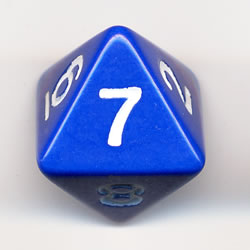An eight-sided dice

Probability Level 5An eight-sided dice is rolled 3 times.
If the number obtained on the third roll is equal to the sum of the numbers obtained on the first roll and second roll, what is the probability that at least one of the rolls was a 2?

If the probability can be expressed as $\dfrac ab$, where $a$ and $b$ are coprime positive integers, find $a+b$.

×